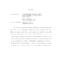## Measurement of the W Boson Mass and Width Using a Novel Recoil Model2009
##### Authors
Wetstein, Matthew Joseph
This dissertation presents a direct measurement of the W boson mass (M_W) and decay width (&Gamma_W) in 1 fb^(-1) of W &rarr e &nu$collider data at D0 using a novel method to model the hadronic recoil. The mass is extracted from fits to the transverse mass M_T, p_T(e), and MET distributions. The width is extracted from fits to the tail of the M_T distribution. The electron energy measurement is simulated using a parameterized model, and the recoil is modeled using a new technique by which Z recoils are chosen from a data library to match the p_T and direction of each generated W boson. We measure the the W boson mass to be M_W = 80.4035 &plusmn 0.024 (stat) &plusmn 0.039 (syst) from the M_T, M_W = 80.4165 &plusmn 0.027 (stat) &plusmn 0.038 (syst) from the p_T(e), and M_W = 80.4025 &plusmn 0.023 (stat) &plusmn 0.043 (syst) from the MET distributions. &Gamma_W is measured to be &Gamma_W=2.025 &plusmn 0.038 (stat) &plusmn 0.061 (syst)$ GeV.